Question

# A square, single-turn wire loop ! - 1.00 cm on a side is placed inside a...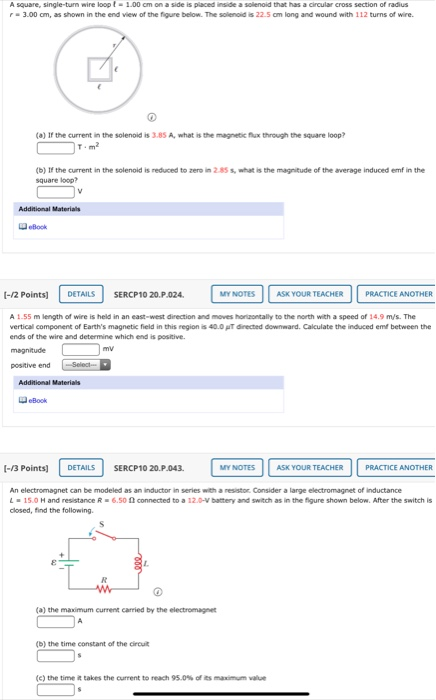A square, single-turn wire loop ! - 1.00 cm on a side is placed inside a solenoid that has a circular cross section of radius - 3.00 cm, as shown in the end view of the figure below. The solenoid is 22.5 cm long and wound with 112 turns of wire. (a) If the current in the solenoid is 3.85 A, what is the magnetic flux through the square loop? (b) If the current in the solenoid is reduced to zero in 285 s, what is the magnitude of the average Induced emf in the square foop? Additional Materials eBook (-12 points) DETAILS SERCP10 20.P.024 MY NOTES ASK YOUR TEACHER PRACTICE ANOTHER A 1.55 m length of wire is held in an east-west direction and moves horizontally to the north with a speed of 14.9 m/s. The vertical component of Earth's magnetic field in this region is 40.0 T directed downward. Calculate the induced emf between the ends of the wire and determine which end is positive. magnitude mv positive end Sinh Additional Materials Book [-/3 Points] DETAILS SERCP10 20.P.043 MY NOTES ASK YOUR TEACHER PRACTICE ANOTHER An electromagnet can be modeled as an inductor in series with a resistor Consider a large electromagnet of Inductance 1 - 15.0 H and resistance R6.50 connected to a 12.0-V battery and switch as in the figure shown below. After the switch is closed, find the following (a) the maximum current carried by the electromagnet (b) the time constant of the circuit (c) the time it takes the current to reach 95.0% of its maximum value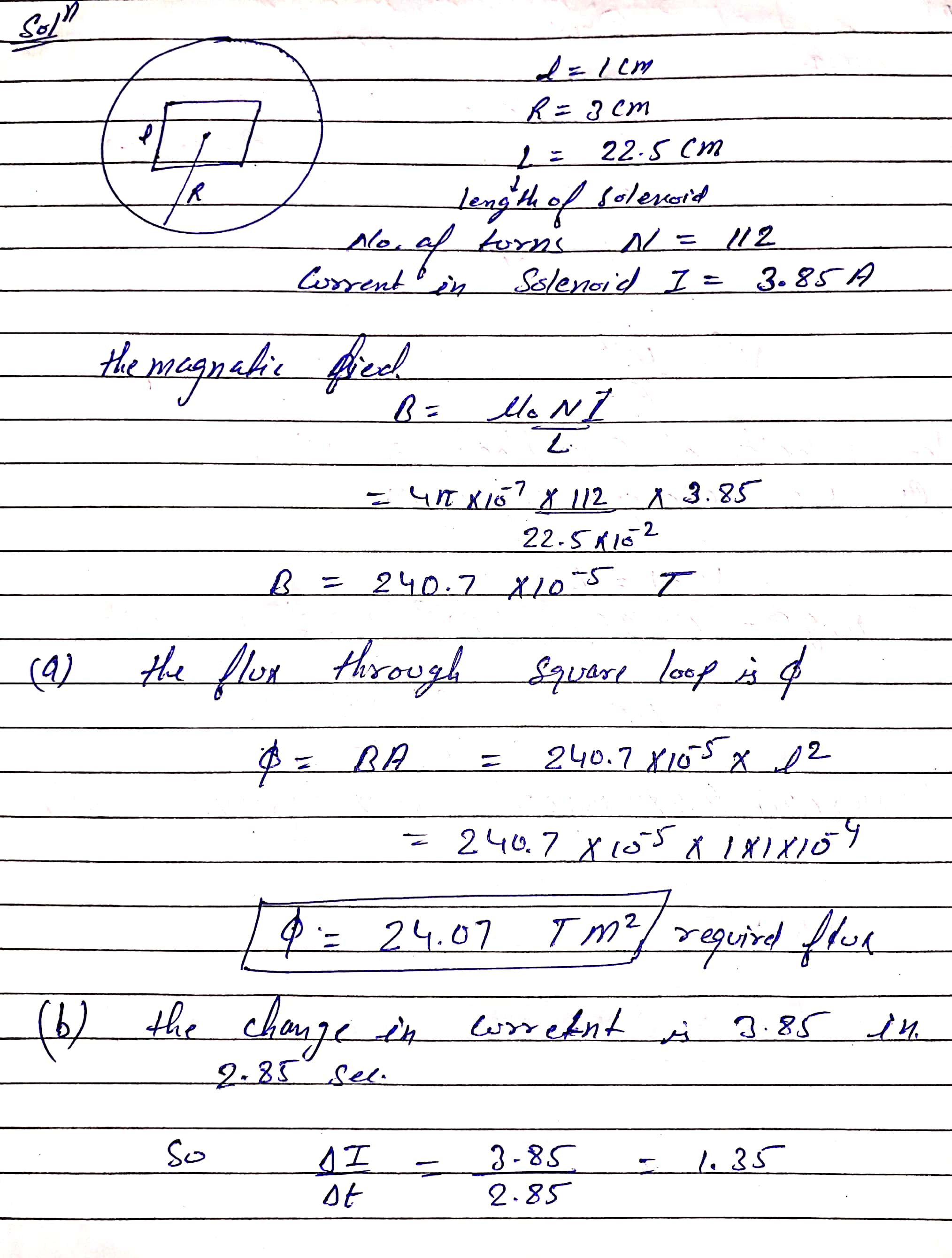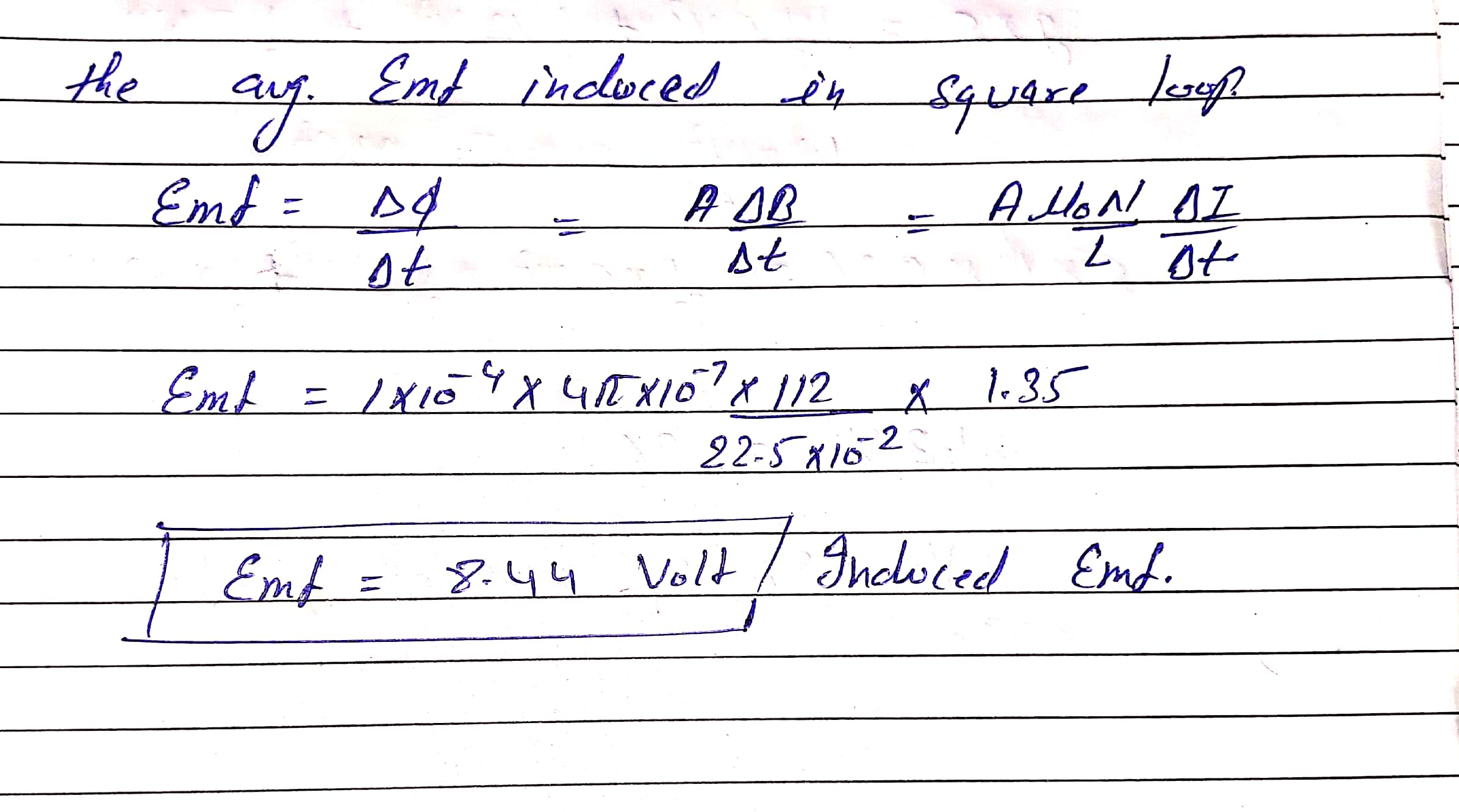If you have any doubt then mention in the comment

#### Earn Coins

Coins can be redeemed for fabulous gifts.

Similar Homework Help Questions
• ### A square, single-turn wire loop ℓ = 1.00 cm on a side is placed inside a...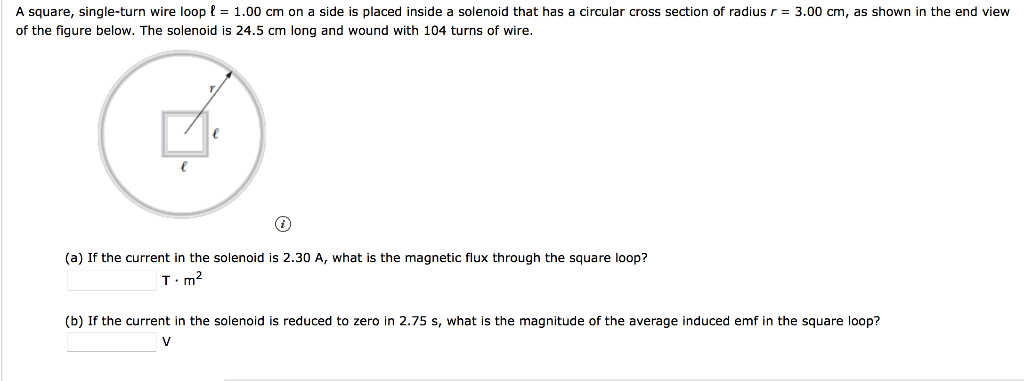A square, single-turn wire loop ℓ = 1.00 cm on a side is placed inside a solenoid that has a circular cross section of radius r = 3.00 cm, as shown in the end view of the figure below. The solenoid is 24.5 cm long and wound with 104 turns of wire. (a) If the current in the solenoid is 2.30 A, what is the magnetic flux through the square loop? T · m2 (b) If the current in the...

• ### A square, single-turn wire loop ℓ = 1.00 cm on a side is placed inside a...

A square, single-turn wire loop ℓ = 1.00 cm on a side is placed inside a solenoid that has a circular cross section of radius r = 3.00 cm, as shown in the end view of the figure below. The solenoid is 24.0 cm long and wound with 122 turns of wire. (a) If the current in the solenoid is 2.35 A, what is the magnetic flux through the square loop? T · m2 (b) If the current in the...

• ### A square, single-turn wire loop1.00 cm on a side is placed inside a solenoid is 23.0...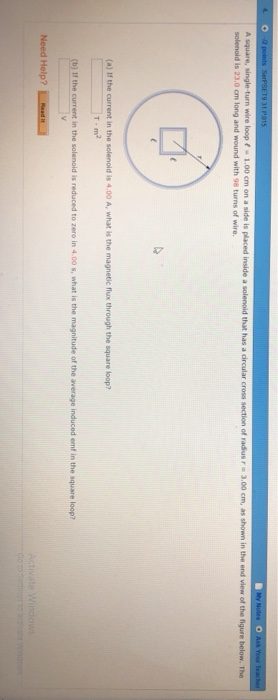A square, single-turn wire loop1.00 cm on a side is placed inside a solenoid is 23.0 cm long and wound with 98 turns of wire that has a circular cross of radius r 3.00 cm, as shown in the end view of the figure below. The (a) If the current in the solenoid is 4.00 A, what is the magnetic flux through the square loop? T-m2 lǐ the current in the solen old is reduced to zero in 4.00 s,...

• ### A square loop with a single turn, with side L= 0.45cm is placed inside a solenoid...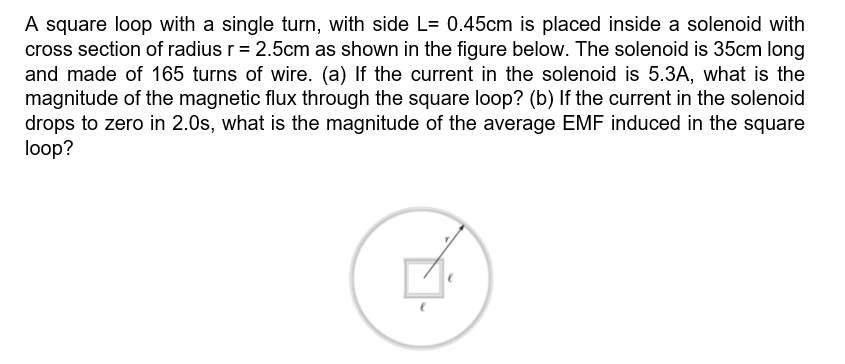A square loop with a single turn, with side L= 0.45cm is placed inside a solenoid with cross section of radius r = 2.5cm as shown in the figure below. The solenoid is 35cm long and made of 165 turns of wire. (a) If the current in the solenoid is 5.3A, what is the magnitude of the magnetic flux through the square loop? (b) If the current in the solenoid drops to zero in 2.0s, what is the magnitude of...

• ### A square, single-turn wire coil L = 1.52 cm on a side is placedinside a...

A square, single-turn wire coil L = 1.52 cm on a side is placed inside a solenoid that has a circular cross-section of radius r = 2.79 cm, as shown in the figure below.The solenoid is 18.0 cm long and wound with 107 turns of wire. If the current in the solenoid is 3.38 A, find the flux through the coil.If the current in the solenoid is reduced to zero in 3.37 s, calculate the magnitude of the average induced...

• ### An electron is moving at a speed of 2.40 109 m/s in a circular path of...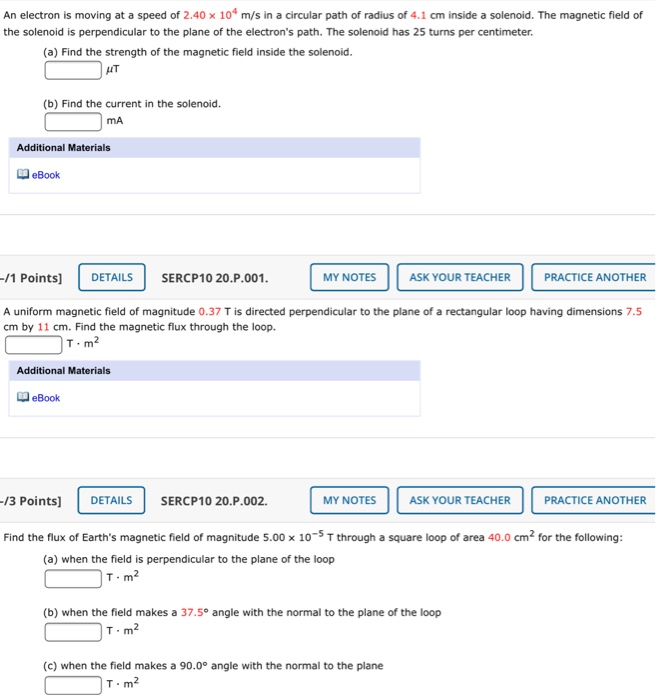An electron is moving at a speed of 2.40 109 m/s in a circular path of radius of 4.1 cm inside a solenoid. The magnetic field of the solenoid is perpendicular to the plane of the electron's path. The solenoid has 25 turns per centimeter. (a) Find the strength of the magnetic field inside the solenoid. AT (b) Find the current in the solenoid. mA Additional Materials eBook -/1 Points) DETAILS SERCP10 20.P.001. MY NOTES ASK YOUR TEACHER PRACTICE ANOTHER...

• ### A square conducting loop with side length a = 1.25 cm is placed at the center...

A square conducting loop with side length a = 1.25 cm is placed at the center of a solenoid 40.0 cm long with a current of 4.60 A flowing through its 470 turns, and it is aligned so that the plane of the loop is perpendicular to the long axis of the solenoid. The radius of the solenoid is 5.00 cm. (a) What is the magnetic flux through the loop? Wb (b) What is the magnitude of the average emf...

• ### 1. A square loop of wire with one side of a and resistance R is placed...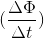1. A square loop of wire with one side of a and resistance R is placed in a uniform magnetic field of strength B. The field vanishes and results an induced current I in the loop. ( a) a) What is the rate of change of magnetic flux in terms of I and R? (b) Magnetic field B (on the picture above) was equal to 0.5 T before it vanished. If the duration of switch is 0.15 sec and the...

• ### A square coil of wire of side 3.80 cm is placed in a uniform magnetic field...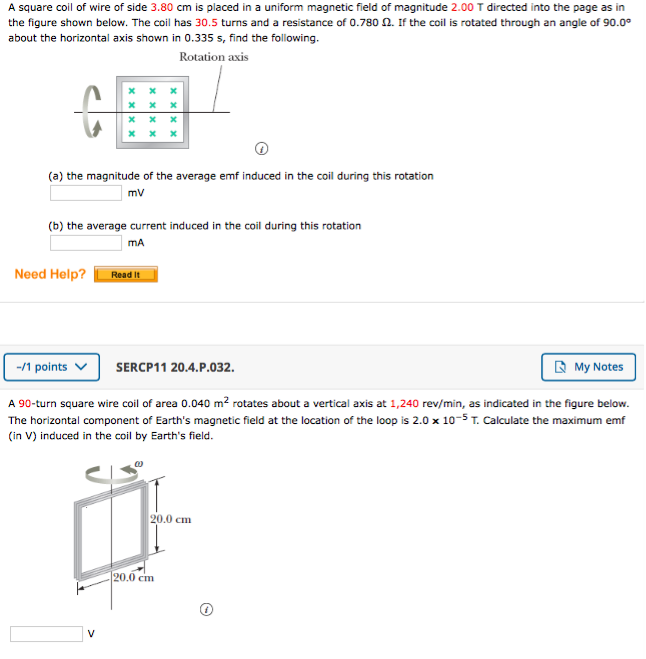A square coil of wire of side 3.80 cm is placed in a uniform magnetic field of magnitude 2.00 T directed into the page as in the figure shown below. The coil has 30.5 turns and a resistance of 0.780 2. If the coil is rotated through an angle of 90.00 about the horizontal axis shown in 0.335 s, find the following. Rotation axis X X X (a) the magnitude of the average emf induced in the coil during this...

• ### A single-turn square loop of wire, 2.00 cm on each edge, carries a clockwise current of...

A single-turn square loop of wire, 2.00 cm on each edge, carries a clockwise current of 0.230 A. The loop is inside a solenoid, with the plane of the loop perpendicular to the magnetic field of the solenoid. The solenoid has 30.0 turns/cm and carries a clockwise current of 15.0 A. (a) Find the force on each side of the loop. magnitude µN direction Correct: Your answer is correct. (b) Find the magnitude of the torque acting on the loop....